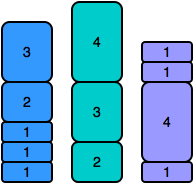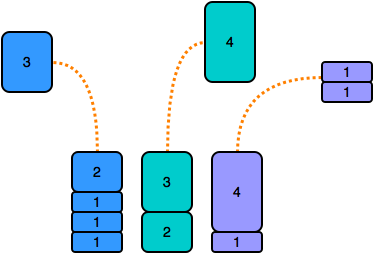# Equal Stacks HackerRank SolutionEqual Stacks HackerRank Solution

In this Equal Stacks HackerRank solution, You have three stacks of cylinders where each cylinder has the same diameter, but they may vary in height. You can change the height of a stack by removing and discarding its topmost cylinder any number of times.

Find the maximum possible height of the stacks such that all of the stacks are exactly the same height. This means you must remove zero or more cylinders from the top of zero or more of the three stacks until they are all the same height, then return the height.

Example

There are  and  cylinders in the three stacks, with their heights in the three arrays. Remove the top 2 cylinders from  (heights = [1, 2]) and from  (heights = [1, 1]) so that the three stacks all are 2 units tall. Return  as the answer.

Note: An empty stack is still a stack.

Function Description

Complete the equalStacks function in the editor below.

equalStacks has the following parameters:

• int h1[n1]: the first array of heights
• int h2[n2]: the second array of heights
• int h3[n3]: the third array of heights

Returns

• int: the height of the stacks when they are equalized

Input Format

The first line contains three space-separated integers, , , and , the numbers of cylinders in stacks , , and . The subsequent lines describe the respective heights of each cylinder in a stack from top to bottom:

• The second line contains  space-separated integers, the cylinder heights in stack . The first element is the top cylinder of the stack.
• The third line contains  space-separated integers, the cylinder heights in stack . The first element is the top cylinder of the stack.
• The fourth line contains  space-separated integers, the cylinder heights in stack . The first element is the top cylinder of the stack.

Constraints

Sample Input

STDIN       Function
-----       --------
5 3 4       h1[] size n1 = 5, h2[] size n2 = 3, h3[] size n3 = 4
3 2 1 1 1   h1 = [3, 2, 1, 1, 1]
4 3 2       h2 = [4, 3, 2]
1 1 4 1     h3 = [1, 1, 4, 1]


Sample Output

5


Explanation

Initially, the stacks look like this:To equalize thier heights, remove the first cylinder from stacks  and , and then remove the top two cylinders from stack  (shown below).The stack heights are reduced as follows:

All three stacks now have , the value to return.

## Equal Stacks HackerRank solution

I will Provide solution in Multiple programming languages for you. If you are not able to find the code in required language then please share in comments so that our team can help you.

### Problem Solution in Python

def equalStacks(h1, h2, h3):
stacks = [h1,h2,h3]
heights = [sum(h1), sum(h2), sum(h3)]
indexes = [0,0,0]
while not all(v == heights for v in heights):
max_idx = heights.index(max(heights))
heights[max_idx] -= stacks[max_idx][indexes[max_idx]]
indexes[max_idx] += 1

return heights

### Problem Solution in C++

int equalStacks(vector<int> h1, vector<int> h2, vector<int> h3) {
vector<int> hsum(3);
hsum = accumulate(h1.begin(), h1.end(), 0);
hsum = accumulate(h2.begin(), h2.end(), 0);
hsum = accumulate(h3.begin(), h3.end(), 0);
while (hsum != hsum || hsum != hsum || hsum != hsum) {
//find largest
int maximum = max(hsum, max(hsum, hsum));
if (hsum == maximum) {
hsum -= h1.front();
h1.erase(h1.begin());
} else if (hsum == maximum) {
hsum -= h2.front();
h2.erase(h2.begin());
} else {
hsum -= h3.front();
h3.erase(h3.begin());
}
cout << hsum << " " << hsum << " " << hsum << endl;
}
return hsum;
}

### Problem Solution in C#

public static int equalStacks(List<int> h1, List<int> h2, List<int> h3)
{
var sums = new Dictionary<List<int>, int>
{
[h1] = h1.Sum(),
[h2] = h2.Sum(),
[h3] = h3.Sum()
};

while (!sums.All(x => x.Value == sums.First().Value))
{
var largest = sums.OrderByDescending(x => x.Value).First();
sums[largest.Key] = largest.Value - largest.Key;
largest.Key.RemoveAt(0);
}

return sums.First().Key.Sum();
}

### Problem Solution in JavaScript

function equalStacks(h1, h2, h3) {
let h = [h1, h2, h3]
let totals = h.map(h => h.reduce((p, c) => p + c))
let max
while (totals.some((e) => e !== (max = Math.max(...totals))))  {
if (Math.min(...totals) === 0) return 0
const i = totals.indexOf(max)
totals[i] -= h[i].shift()
}
}